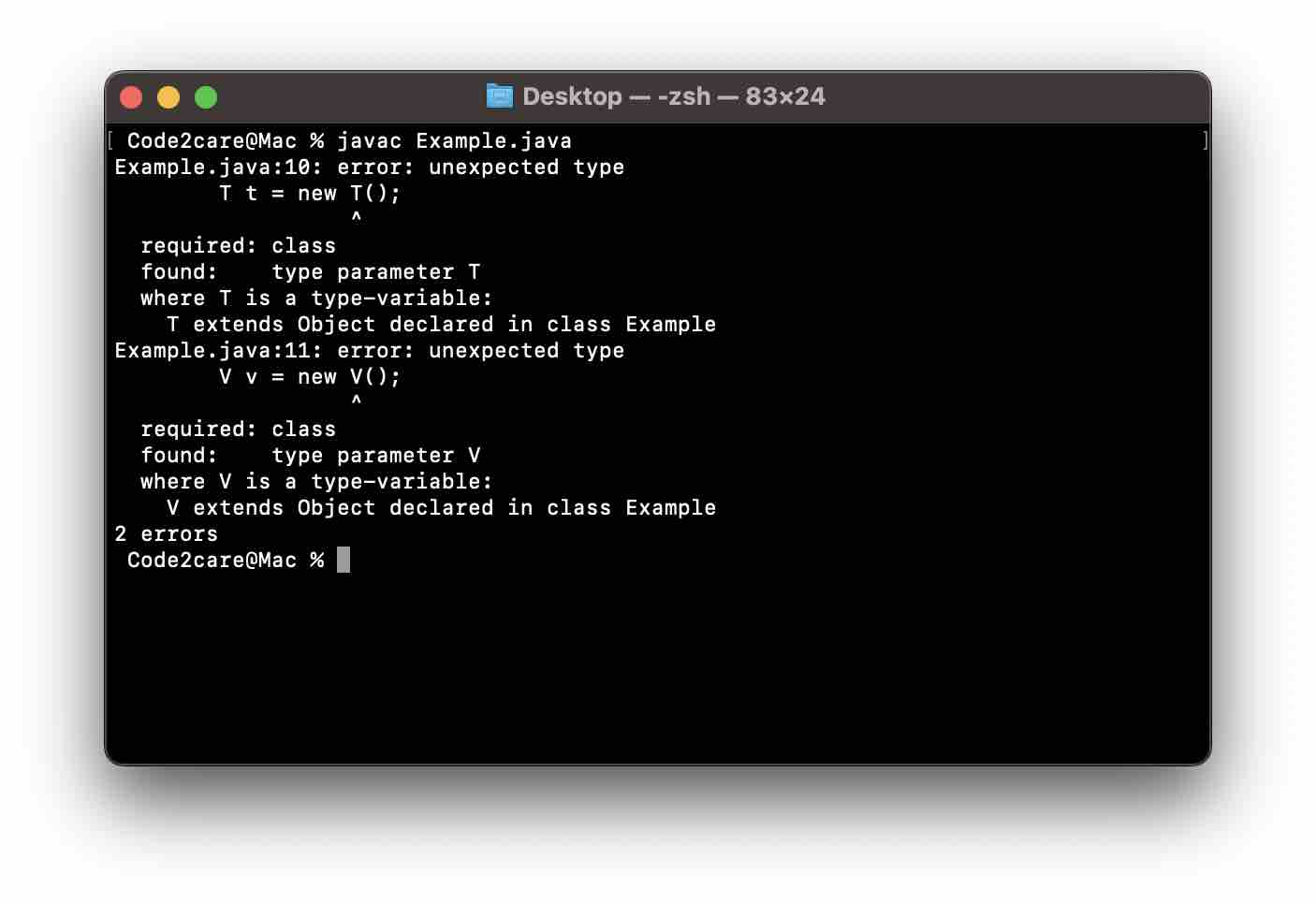# Fix Generics: error unexpected type required: class found: type parameter

Code:
``````package org.code2care.generics;

public class Example<T,V> {

public static void main(String[] args) {
System.out.println("Generics Example!");
}

public void method() {
T t = new T();
V v = new V();
}
}``````
Compilation Error:
``````Code2care@Mac % javac Example.java
Example.java:10: error: unexpected type
T t = new T();
^
required: class
found:    type parameter T
where T is a type-variable:
T extends Object declared in class Example

Example.java:11: error: unexpected type
V v = new V();
^
required: class
found:    type parameter V
where V is a type-variable:
V extends Object declared in class Example
2 errors``````

This is a violation of the rules of dealing with Generic types in Java. You cannot create an instance of generic type parameters.

Hence the compilation error clearly states that it was expecting a class type but a type parameter was provided.Solution:

The ideal way would be to pass the type parameters as parameters to your method.

``````package org.code2care.generics;

public class Example<K,V> {

public static void main(String[] args) {
Example<String,Integer> example = new Example<>();
example.method("Sam",20);
}

public void method(K k, V v) {
System.out.println(k);
System.out.println(v);
}
}``````
-

Have Questions? Post them here!